Students must start practicing the questions from CBSE Sample Papers for Class 10 Maths with Solutions Set 5 are designed as per the revised syllabus.

## CBSE Sample Papers for Class 10 Maths Standard Set 5 with Solutions

Time Allowed: 3 Hours
Maximum Marks: 80

General Instructions:

1. This Question Paper has 5 Sections A-E.
2. Section A has 20 MCQs carrying 1 mark each
3. Section B has 5 questions carrying 02 marks each.
4. Section C has 6 questions carrying 03 marks each.
5. Section D has 4 questions carrying 05 marks each.
6. Section E has 3 case based integrated units of assessment (04 marks each) with sub-parts of the values of 1, 1 and 2 marks each respectively.
7. All Questions are compulsory. However, an internal choice in 2 Qs of 5 marks, 2 Qs of 3 marks and 2 Questions of 2 marks has been provided. An internal choice has been provided in the 2 marks questions of Section E
8. Draw neat figures wherever required. Take π =22/7 wherever required if not stated.

Section – A
(Section A consists of 20 questions of 1 mark each.)

Question 1.
The LCM. of ( 23 K 3 x 5) and (24 x 5 x 7) is: (1)
(a) 1540
(b) 1680
(c) 1640
(d) 1200
(b) 1680

Explanation:
LCM of (23 × 3 × 5) and (24 × 5 × 7)
LCM = 24 × 3 × 5 × 7 = 1,680

Question 2.
A quadratic polynomial, whose zeros are -3 and 4 is: (1)
(a) x2 + x + 5
(b) x2 – x + 6
(c) x2 – x – 12
(d) x2 + 2x – 6
(c) x2 – x – 12

Explanation:
A quadratic polynomial with zeros -3 and 4 is:
(x + 3)(x – 4)
i.e. x2 – x – 12

Question 3.
The 11th term of the A.P. $$\sqrt{2}$$, 3$$\sqrt{2}$$, 5$$\sqrt{2}$$, ……… is: (1)
(a) 21$$\sqrt{2}$$
(b) 20$$\sqrt{2}$$
(c) 17$$\sqrt{2}$$
(d) 22$$\sqrt{2}$$
(a) 21$$\sqrt{2}$$

Explanation:
Here, 11th term, a11 = a + 10d
= $$\sqrt{2}$$ + 10(2$$\sqrt{2}$$) = 21$$\sqrt{2}$$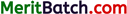Question 4.
The nature of the quadratic equation 2x2 + x + 4 = 0 is: (1)
(a) no real roots
(b) real roots
(c) equal roots
(d) None of these
(a) no real roots

Explanation:
For the equation 2x2 + x + 4 = 0
D = b2 – 4ac = 1 – 32 = -31
The given equation has no real roots.

Question 5.
The 2nd term of the AP, if its Sn = n2 + 2n is: (1)
(a) 4
(b) 2
(c) 8
(d) 5
(d) 5

Explanation:
Here, a2 = S2 – S1 = (22 + 2 × 2) – (12 + 2 × 1)
= (4 + 4) – (1 + 2) = 5

Question 6.
The roots of x + $$\frac{1}{x}$$ = 2 are: (1)
(a) 5,1
(b) 2, 3
(c) 1,1
(d) 4, -2
(c) 1. 1

Explanation:
The equation is, x + $$\frac{1}{x}$$ = 2
x2 – 2x + 1 = 0, (x – 1)2 = 0
So, roots are 1, 1.

Question 7.
A pair of linear equations, which has the unique solution x = -1, y = 3, is: (1)
(a) x – y = -4, x + 2y = 5
(b) 2x + y = 0, x – 3y = 7
(c) x + y = 4, x + y = 7
(d) x + y = 0, x – y = 2
(a) x – y = – 4, x + 2y = 5

Explanation:
As x = -1, y = 3 is a point.
So many lines can pass through a point.
Therefore, infinitely many pairs are possible in (a)
x – y = – 4
– 1 – 3 = – 4
– 4 = – 4

x + 2y = 5
-1+ 2 × 3 = 5
-1 + 6 = 5
5 = 5Question 8.
If the distance between the points (4, p) and (1, 0) is 5 units, then the value of p is: (1)
(a) 3
(b) ± 4
(c) 5
(d) – 3
(b) ±4

Explanation:
Here, $$\sqrt{(4 – 1)^2+(p – 0)^2}$$ = 5
⇒ 9 + p2 = 25
⇒ p = ±4

Question 9.
A tangent PQ at a point P of a circle of radius 5 cm meets a line through the centre O at a point Q so that OQ = 12 cm. Then, the length of PQ is: (1)
(a) $$\sqrt{119}$$ cm
(b) 16 cm
(c) 15 cm
(d) $$\sqrt{211}$$ cm
(a) $$\sqrt{119}$$ cm

Explanation:
Since OP ⊥ PQ,PQ = $$\sqrt{(OQ)^2+(OP)^2}$$ = $$\sqrt{(12)^2+(5)^2}$$
= $$\sqrt{144+25}$$ = $$\sqrt{119}$$ cm.

Question 10.
The distance between the points (0, 6) and (0, -2) is: (1)
(a) 5 units
(b) 6 units
(c) 8 units
(d) 3 units
(c) 8 units

Explanation:
Given, points are P(0, 6) and Q(0, -2)
By distance formula, distance between PQ is
PQ = $$\sqrt{(0-0)^2+(-2-6)^2}$$
= $$\sqrt{0^2+(-8)^2}$$
=$$\sqrt{0+64}$$
= 8 units

Question 11.
For the distribution given below, the median class is: (1)

 Marks Frequency Below 10 3 Below 20 12 Below 30 27 Below 40 57 Below 50 75 Below 60 80

(a) 20 – 30
(b) 60 – 70
(c) 30 – 40
(d) 40-50
(c) 30 – 40

Explanation:
Here, $$\frac{N}{2}$$ = $$\frac{80}{2}$$ = 40
Then, median class is 30-40.Question 12.
The value of (1 + cos A)(1 – cos A) cosec2 A is: (1)
(a) 0
(b) $$\frac{1}{2}$$
(c) 1
(d) $$\frac{\sqrt{3}}{2}$$
(c) 1

Explanation:
Here,
(1 + cos A) (1 – cosA) cosec2A
= (1 – cos2 A) cosec2 A
= sin2 A cosec2 A
[sin2 A + cos2 A = 1]
= 1

Question 13.
Sarita buys a fish from a shop for her aquarium. The shopkeeper takes out a fish at random from a tank containing 10 male fishes and 12 female fishes. What is the probability that the fish taken out is a female fish? (1)
(a) $$\frac{3}{22}$$
(b) $$\frac{2}{22}$$
(c) $$\frac{5}{11}$$
(d) $$\frac{6}{11}$$
(d) $$\frac{6}{11}$$

Explanation:
Total number of fishes =10+12 = 22
Probability (female fish) = $$\frac{\text { Total number of female fishes }}{\text { Total number of fishes }}$$
= $$\frac{12}{22}$$
= $$\frac{6}{11}$$

Question 14.
Write the formula used for calculating the median of a grouped frequency distribution.
(a) $$l+\frac{\frac{N}{2}-c f}{f} \times h$$
(b) $$\frac{N}{2}$$
(c) $$\frac{N}{2}(l+c f)$$
(d) $$\frac{l+c f}{f}$$
(a) $$l+\frac{\frac{N}{2}-c f}{f} \times h$$

Explanation:
Median = $$l+\frac{\frac{N}{2}-c f}{f} \times h$$

Question 15.
The maximum value of $$\frac{1}{cosec θ}$$ is:
(a) $$\frac{\sqrt{3}}{2}$$
(b) $$\frac{1}{\sqrt{2}}$$
(c) 0
(d) 1
(d) 1

Explanation:
Since $$\frac{1}{cosec θ}$$ = sin θ
And maximum value of sin θ is 1 i.e, when
θ = 90°

Question 16.
Find the number, if eight times of it is added to its square , the sum so obtained is -16. (1)
(a) – 4
(b) 5
(c) 3
(d) 2
(a) -4

Explanation:
Let the number be x
Then, according to the question
8x + x2 = -16
⇒ x2 + 8x + 16 = 0
⇒ x2 + 4x + 4x + 16 = 0
⇒ (x + 4) (x + 4) = 0
⇒ x = -4, -4
Hence, the number is -4.Question 17.
Write the formula used for calculating the mode of a grouped frequency distribution. (1)
(a) $$l+\frac{f_1-f_0}{h}$$
(b) $$\frac{f_1-f_0}{2 f-f_0} \times h$$
(c) $$\frac{f_1-f_0}{2} \times h$$
(d) $$l+\left(\frac{f_1-f_0}{2 f_1-f_0-f_2}\right) \times h$$
(d) $$l+\left(\frac{f_1-f_0}{2 f_1-f_0-f_2}\right) \times h$$

Explanation:
Since Median = $$l+\left(\frac{f_1-f_0}{2 f_1-f_0-f_2}\right) \times h$$

Question 18.
If tan θ + cot θ = 2, then the value of tan2 θ + cot2 θ is:
(a) 2
(b) 3
(c) 4
(d) 5
(a) 2

Explanation:
Given, tan θ + cot θ = 2 = 2
Then, on squaring both sides, we get
tan2 θ + cot2 θ + 2 tan θ cot θ = 4
⇒ tan2 θ + cot2 θ = 4 – 2 tan θ cot θ
⇒ tan2 θ + cot2 θ = 4 – 2 tan θ × $$\frac{1}{tan θ}$$,
=4 – 2 = 2

DIRECTION: In the question number 19 and 20, a statement of assertion (A) is followed by a statement of reason (R).
Choose the correct option as:
(a) Both assertion (A) and reason (R) are true and reason (R) is the correct explanation of assertion (A)
(b) Both assertion (A) and reason (R) are true and reason (R) is not the correct explanation of assertion (A)
(c) Assertion (A) is true but reason (R) is false.
(d) Assertion (A) is false but reason (R) is true.

Question 19.
Statement A (Assertion): If a die is thrown, the probability of getting a number less than 3 and greater than 2 is zero.
Statement R (Reason): Probability of an impossible event is zero. (1)
(a) Both assertion (A) and reason (R) are true and reason (R) is the correct explanation of assertion (A)

Explanation:
The probability of getting a number less than 3 and greater than 2 is 0. Event given in Assertion is an impossible event

Question 20.
Statement A (Assertion): The radii of two cones are in the ratio 2:3 and their volumes in the ratio 1:3. Then the ratio of their heights is 3:2.
Statement R (Reason): The Volume of the cone = $$\frac{1}{2}$$ πr2h
(d) Assertion (A) is false but reason (R) is true.

Explanation:
Ratio of volumeSection – B
(Section B consists of 5 questions of 2 marks each.)

Question 21.
Assuming that $$\sqrt{2}$$ is irrational, show that 5$$\sqrt{2}$$ is an irrational number. (2)
Let 5$$\sqrt{2}$$ be rational. Then,
5$$\sqrt{2}$$ = $$\frac{p}{q}$$ , where p and q are co-prime.
⇒ $$\sqrt{2}$$ = $$\frac{p}{5q}$$
Here, $$\frac{p}{5q}$$ is rational, which implies $$\sqrt{2}$$ is rational which is a contradiction, as $$\sqrt{2}$$ is irrational.
∴ 5$$\sqrt{2}$$ is an irrational number.

Question 22.
Find the greatest number that divides 338 and 59 and leaves remainders of 2 and 5 respectively.
OR
Find the coordinates of the point, where the perpendicular bisector of the line segment joining the points A(l,5) and B(4,6) cuts the y-axis. (2)
Since 338 and 59 divided by the required number leave remainder of 2 and 5 respectively.
338 – 2 = 336; 59 – 5 = 54
are completely divisible by the number Now, we have to find HCF of 336 and 54.336 = 24 × 3 × 7
54 = 2 × 33
HCF = 2 × 3 = 6
Hence, the required number is 6.
OR
Since, the ⊥r bisector of line segment AB bisect the segment AB,
i.e., ⊥r bisector of line segment AB passes through the mid-point of AB.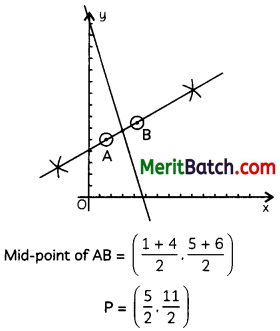Question 23.
Prove that the lengths of tangents drawn from an external point to a circle are equal. (2)
Let AP and AQ be the two tangents drawn to the circle form the extranal point A.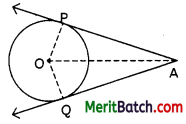We need to show that AP = AQ.
Join OA, OP and OQ.
Consider ∆OPA and ∆OQA.
Here, OQ = OP (radii of the circle)
OA = OA (common)
∠OPA = ∠OQA [Each 90°]
So, ∆OPA ≅ ∆OQA
⇒ PA = QA or AP = AQ.

Question 24.
Find the angle of elevation of the sun when the shadow of a pole ‘h’ metres high is $$\sqrt{3}$$ h metres long. (2)
Here, AB is a pole of height ‘h’m and its shadow BC of length $$\sqrt{3}$$h m Let the angle of elevation be θ.Then,
tan θ = $$\frac{AB}{BC}$$ = $$\frac{h}{\sqrt{3}h}$$
tan θ = $$\frac{1}{\sqrt{3}}$$
⇒ tan θ = tan 30°
θ = 30°Question 25.
How many terms of AP : 18, 16, 14, ……. make the sum zero?
OR
Find the volume of the largest right circular cone that can be cut out of a cube whose edge is 7 cm. (2)
Let n term of the given AP make the sum zero.
Then,
$$\frac{n}{2}$$[18 × 2+(n-1)(-2] =0
⇒ 36 – 2(n – 1) = 0
⇒ 2n – 2 = 36
⇒ 2n = 38
⇒ n = 19
OR
The base radius and the vertical height of the largest cone that can be carved out of a cube are $$\frac{7}{2}$$ cm and 7 cm respectively.
So, volume =$$\frac{1}{3} \pi\left(\frac{7}{2}\right)^2 (7) \mathrm{cm}^2$$
= $$\frac{1}{3} \times \frac{22}{7} \times \frac{7}{2} \times \frac{7}{2} \times 7 \mathrm{~cm}^2$$
= 89.83 cm2

Section – C
(Section C consists of 6 questions of 3 marks each.)

Question 26.
Determine the zeros of the polynomial p(x) = x3 – 2x2. Also verify the relationship between the zeros and the coefficient. (3)
Here, p(x) = x3 – 2x2
For calculating zeros, put p (x) = 0
x2 – 2x2 = 0
⇒ x2(x – 2) = 0
⇒ x = 0, 0, 2
The required zeros are 0, 0 and 2.

Here, a = 0, p = 0 and y = 2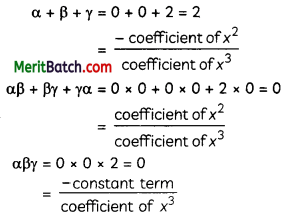Question 27.
A sum of ₹ 250 was divided equally among a certain number of children. If there were 25 more children, each would have received 50 paise less. Find the number of children.
OR
The centre of a circle is C(2a, a, -7). Find the values of‘a’ if the circle passes through the point P(11, -9) and has diameter 10$$\sqrt{3}$$ units. (3)
Let the number of children be ‘x’. Then,
amount received by each child = ₹ ($$\frac{250}{x}$$)
When there are (x + 25) children,
amount received by each child = ₹ ($$\frac{250}{x + 25}$$)
As per the question,
$$\frac{250}{x}$$ – $$\frac{250}{x + 25}$$ = $$\frac{1}{2}$$
⇒ x(x + 25) = 12500
⇒ x2 + 25x – 12500 = 0
⇒ x2+125x-100x- 12500 =0
⇒ x(x + 125) -100 (x + 125) = 0
⇒ (x + 125) (x – 100) = 0
⇒ x+125 = 0 or x-100 = 0
⇒ x = 100
(∵ x ≠ 125)
Hence, there were 100 children in all
OR
Let the given point P(x1, yi) = (11, -9) lie on a circle with centre C(x2, yi) = (2a, a – 7) and radius ‘r’.
Then, PC = r …. (i)
Given, that the diameter of circle is 10$$\sqrt{2}$$ units.Hence, the value of‘a’ are 5 and $$\frac{3}{5}$$.Question 28.
In the figure, PQ and RS are the common tangents of two circles intersecting at O.Prove that: PQ = RS. (3)
From the figure, OP = OR and OS = OQ
⇒ PQ = PO + OQ
= OR + OS
= RS

Question 29.
In ∆ABC, ∠A is acute. BD and CE are perpendiculars on AC and AB respectively. Prove that AB × AE = AC × AD. (3)∠A = ∠A [common angle]
So, by AA similarity criterion,
⇒ $$\frac{AE}{AD}$$ = $$\frac{AC}{AB}$$
⇒ AB × AE = AC × AD

Question 30.
If x = a cos3 θ and y = b sin2 θ, then prove that $$\left(\frac{x}{a}\right)^{2 / 3}+\left(\frac{y}{a}\right)^{2 / 3}$$. (3)
$$\left(\frac{x}{a}\right)^{2 / 3}+\left(\frac{y}{a}\right)^{2 / 3}$$ = $$\left(\frac{a \cos ^3 \theta}{a}\right)^{\frac{2}{3}}+\left(\frac{b \cos ^3 \theta}{b}\right)^{\frac{2}{3}}$$
= $$\cos ^2 \theta+\sin ^2 \theta$$
= 1

Question 31.
For the following frequency distribution, find the median marks:

 Marks Number of students 0-20 7 20-40 12 40-60 23 60-80 18 80-100 10

OR
I toss three coins together. The possible outcomes are no heads, 1 head, 2 heads and 3 heads. So, I can say that the probability
of no heads is $$\frac{1}{4}$$. What is wrong with this conclusion? (3)Hence, the median of the given data is 54.
OR
Toss 3 coins getting heads in one = $$\frac{1}{2}$$ ,
so in all three = $$\frac{1}{2} \times \frac{1}{2} \times \frac{1}{2}=\frac{1}{8}$$
So outcomes of 1 head is $$\frac{3}{8}$$ and 2 head is $$\frac{3}{8}$$Section – D
(Section D consists of 4 questions of 5 marks each.)

Question 32.
If a line is drawn parallel to one side of a triangle to intersect the other two sides in distinct points, prove that the other two sides are divided in the same ratio. (5)
ABC is a triangle and DE is a line parallel to side BC which cuts AB at D and AC at E.Question 33.
From the top of a building 60 m high, the angle of depression of the top and bottom of a vertical lamp-post are observed to be 30° and 60° respectively. Find the height of the lamp-post, and the distance between the top of building and the top of lamp post.
OR
Prove that $$\frac{1 + sec A – tan A }{1 + sec A + tan A}$$ = $$\frac{1 – sin A}{cos A}$$ (5)
Let ‘h’ metres be the height of the lamp-post and ‘d’ metres be the distance between feet of the lamp post and the building.Then,
From right ABMY, we have: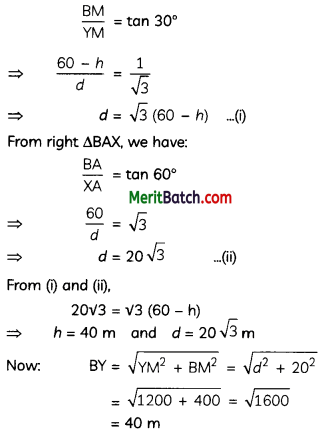Thus, the distance between the top of the building and the top of lamp-post is 40 m.
OR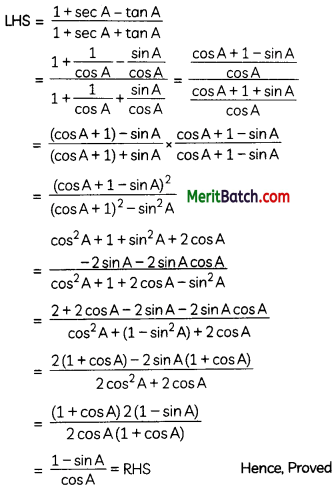Question 34.
Solve the following pair of linear equations by the substitution method.
(A) x + y = 14, x – y = 4
(B) s – t = 3, $$\frac{s}{3}$$ + $$\frac{t}{2}$$ = 6
(C) 3x – y = 3, 9x – 3y = 9
(D) 0.2x + 0.3y = 1.3, 0.4x + 0.5y = 2.3
(E) $$\sqrt{2}$$x + $$\sqrt{3}$$y = 0, $$\sqrt{3}$$x – $$\sqrt{8}$$y = 0
OR
Find the mean and mode for the following frequency distribution: (5)

 Monthly consumption On units) Number of consumers 65-85 4 85-105 5 105-125 13 125-145 20 145-165 14 165-185 8 185-205 4

(A) x + y = 14 …(i)
x – y = 4 …(ii)
x = 4 + y from equation (ii)
Putting this in equation (i), we get
4 + y + y =14
⇒ 2y = 10
⇒ y = 5
Putting value of y in equation (i), we get
⇒ x + 5 = 14
⇒ x = 14 – 5 = 9
Therefore, x = 9 and y = 5

(B) s – t = 3 ……(i)
$$\frac{s}{3}$$ + $$\frac{t}{2}$$ = 6 …….(ii)
Putting s = 3 + t in equation (ii), we get
$$\frac{3+t}{3}$$ = 6
⇒ $$\frac{6+2t+3t}{6}$$ = 6
⇒ 5t + 6 =36
⇒ 5t = 30
⇒ t = 6
Putting value of t in equation (i), we get
⇒ s – 6 = 3
⇒ s =3 + 6 = 9
Therefore, t = 6 and s = 9

(C) 3x – y = 3 …(i)
9x – 3y = 9 …(ii)
Comparing equation 3x – y = 3 with a1x + b1y + C1 = 0 and equation 9x – 3y = 9 with a2x + b2y + C2 = 0,

We get a1 = 3, b1 = – 1, c1 = -3,
a2 = 9, b2 = -3 and c2 = – 9
Here, $$\frac{a_1}{a_2}=\frac{b_1}{b_2}=\frac{c_1}{c_2}$$
Therefore, we have infinite many solutions of x and y.

(D) 0.2x + 0.3y = 1.3 …(i)
0.4x + 0.5y = 2.3 …(ii)
Using equation (i), we can say that
0.2x = 1.3 – 0.3y
⇒ x = $$\frac{1.3-0.3y}{0.2}$$
Putting this in equation (ii), we get
0.4($$\frac{1.3-0.3y}{0.2}$$) + 0.5y = 2.3
⇒ 2.6 – 0.6y + 0.5y = 2.3
⇒ -0.1 y = -0.3
⇒ y = 3
Putting value of y in (i), we get
⇒ 0.2x + 0.3(3) = 1.3
⇒ 0.2x + 0.9 = 1.3
⇒ 0.2x = 0.4
⇒ x = 2
Therefore, x = 2 and y = 3

(E) $$\sqrt{2}$$x + $$\sqrt{3}$$y = 0 ……..(i)
$$\sqrt{3}$$x – $$\sqrt{8}$$y = 0 ……..(ii)

Using equation (i), we can say that
x = $$\frac{\sqrt{3}y}{\sqrt{2}}$$
Putting this in equation (ii), we get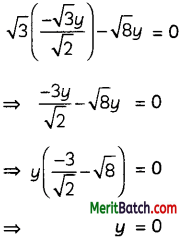Putting value of y in (i), we get x = 0
Therefore, x = 0 and y = 0OR

Mode: Here, modal class is 125 – 145
For this class,
l = 125, f1 = 20, f0 = 13, f2 = 14, h = 20

Mode = $$l+ \frac{f_1-f_0}{2f_1-f_0-f_2} \times h$$
So Mode = $$125+ \frac{20-30}{40-13-14} \times 20$$
= 135.77

Mean: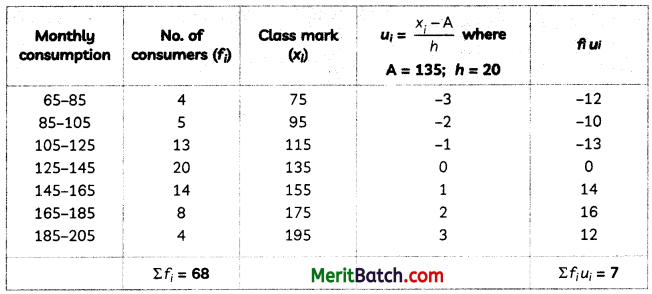Mean = 135 + $$\frac{7}{68}$$ × 20
= 135 + $$\frac{140}{68}$$
= 135 + 2.06
= 137.06

Question 35.
An arc subtends an angle of 60 at the centre of a circle with a radius of 21 cm. find:
(A) the arc’s length.
(B) the area of the sector the arc formed.
(C) the area of the segment that the corresponding chord forms. (5)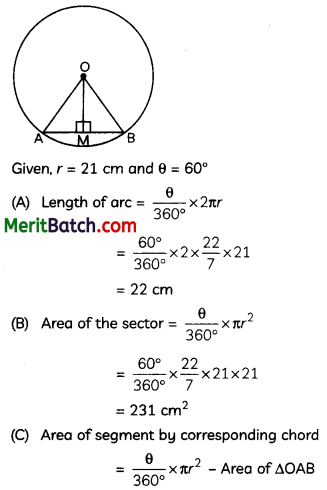⇒ Area of segment = 231 – Area of AOAB …(i)
In right angled triangle OMA and OMB,
OM = OB [Radii of the same circle]
OM = OM [Common]
∴ ∆OMA = ∆OMB [RHS congruency]
.-. AM = BM [By CPCT]
M is the mid-point of AB and ∠AOM = ∠BOM
⇒ ∠AOM = ∠BOM = $$\frac{1}{2}$$ ∠AOB = $$\frac{1}{2}$$ × 60° = 30°
Therefore, in right angled triangle OMA,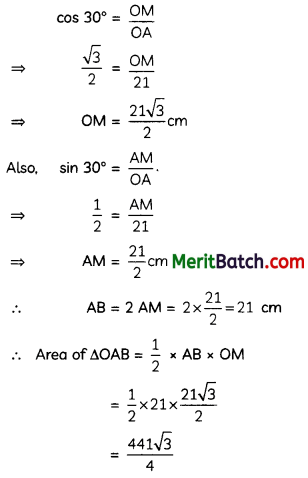Using eq. (i),
Area of segment formed by corresponding chord = (231 – $$\frac{441 \sqrt{3}}{4}$$) cm2Section – E
(Case Study Based Questions)
(Section E consists of 3 questions. All are compulsory.)

Question 36.
‘Origami’ is the art of paper folding, which is often associated with Japanese culture. Gurmeet is trying to learn Origami using paper cutting and folding technique. A square base is sometimes referred to as a “preliminary” base or preliminary fold.Here is a 20 cm x 20 cm square. Gurmeet wants to first cut the squares of integral length from the corners and by folding the flaps along the sides.On the basis of the above information, answer the following questions:
(A) Find the dimensions of the box with maximum volume. (1)
(B) Find the dimensions of the box with minimum volume. (1)
(C) Find the equation relating the size of the square cut out and volume of the box.
OR
How many different sixes of boxes Gurmeet can make? If sides of the square are not integral length then find the number of boxes? (2)
(A) On Calculating the volume of the boxes given in the options. The box with dimension 14 × 14 × 3 has maximum volume as 588.

(B) On Calculating the volume of the boxes given in the option. The box with dimensions 18 × 18 × 1 has minimum volume.

(C) Let the width of square of each side be Y
Then, sides of box are 20 – 2x, 20 – 2x and x
Volume = lbh
= (20 – 2x) (20 – 2x)x
= (400 – 40x – 40x + 4x2)x
= 4x3 – 80x2 + 400x

OR

Different size of squares are = 18 × 18 × 1, 16 × 16 × 2, 14 × 14 × 3, 12 × 12 × 4, 10 × 10 × 5, 8 × 8 × 6….
As the side length of any value could be cut out from the square and it could be infinite in number.Question 37.
The students of a school decided to beautify the school on the Annual day by fixing colourful flags on the straight passage of the schooL They have 27 flags to be fixed at intervals of every 2 m. The flags are stored at the position of the middle most flag.Ruchi was given the responsibility of placing the flags. Ruchi kept her books where the flag were stored She could carry only one flag at a time.
On the basis of the above information, answer the following questions:
(A) What is the position of the middle flag? (1)
(B) Find total distance travelled for placing all the flags. (1)
(C) Find total distance travelled for placing 13 flags on left
OR
Find the maximum distance she travelled carrying a flag. (2)
(A)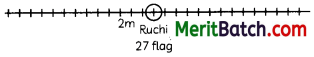There are 27 flags. So the middle most flag is 14th flag.

(B) Total distance travelled = 13 flags on left side + 13 flags on right side
= 364 + 364
= 728 m

(C) For placing first placing she go 2 m and come back 2 m. Then for second flag, she goes 4 m and come back 4 m and so one
….
Distance travelled = 4, 8, 12 ……
Then it forms an A.P. with a = 4, d = 4 and n= 13
Then S13 = $$\frac{13}{2}$$ [8 + 12 × 4]
= $$\frac{13}{2}$$ (56)
= 364 m

OR

The maximum distance that she covered in placing will be the 13th flag on both side
Then,
a13 = a + (n – 1 )d
= 4 + (13 – 1) × 4
= 4 + 48 = 52
∴ From carrying the flag to its position,
the covered distance = $$\frac{52}{2}$$
= 26 mQuestion 38.
Soumya throws a ball upwards, from a rooftop, 80 m above. It will reach a maximum height and then fall back to the ground. The height of the ball from the ground at time ‘t‘ is ‘h’, which is given by,
h = -16t2 + 64t + 80On the basis of the above information, answer the following questions:
(A) What is the height reached by the ball after 1 second? (1)
(B) What is the maximum height reached by the ball? (1)
(C) What are the two possible times to reach the ball at the same height of 128 m?
OR
How long will the ball take to hit the ground? (2)
(A) On the basis of given equation,
h = – 16t2 + 64t + 80
when, t = 1 second
h = – 16(1)2 + 64(1) + 80
= -16 + 144 = 128 m

(B) Rearrange the given equation, by completing the square, we get
h = -16 (t2 – 4t – 5)
= -16[(t – 2)2 – 9]
= – 16(t – 2)2 + 144
Height is maximum, when t = 2
∴ Maximum height = 144 m

(C) Since, h = -16t2 + 16t2 + 80
⇒ 128 = -16t2 + 64t2 + 80
⇒ 16t2 + 64t + 80 – 128 = 0
⇒ 16t2 + 64t – 48 = 0
⇒ t2 – 4t + 3 = 0
⇒ t2 + 3t – t + 3=0
⇒ (t-3) (t-1) = 0
⇒ t = 3, 1
OR
When ball hits the ground, h = 0
-16t2 + 64t + 80 = 0
∴ t2 – 4t – 5 = 0
(t-5)(t+l) = 0
t = 5 or t = -1
Since, time cannot be negative, so the time
= 5 seconds.
= $$\frac{1}{2} \times 21 \times \frac{21 \sqrt{3}}{2}$$